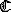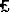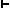## THE ABSOLUTELY STRICT SYSTEMS -- ALTERNATIVE FORMULATIONS

The 'Lemmon-style' bases

WE have been investigating the systems S1° through T in what is essentially the form in which Lewis presented them (with the exception, of course, of and T). But this is not the only way to formulate these systems, nor is it the simplest. Other systems of primitive connectives than K-N-M might be used; most simply, K and N might be replaced by any functionally complete system of connectives for PC, and M might be replaced by L. And yet other connectives might be employed. Instead of L or M, a sign of impossibility or of lack of necessity might be used; in the original formulation of strict implication of Lewis 1918, impossibility was the primitive modal operator. As we shall see, in fact, impossibility along with just material implication provides a complete set of connectives for some of the Lewis-modal systems.

The common base for the systems as we have till now studied them is the system S1°. But S1° itself contains the PC. Is it not possible that the PC itself rather than S1° might be taken as the base for these calculi? It is indeed possible, and as it turns out, perhaps even preferable to do so. As we shall see, formulations of these systems based upon the classical PC are more elegant and economical than those we have seen up to now, and they also permit these logics to be treated as 'tiered' systems, with all the theorems not containing modal symbols provable from the non-modal axioms. Simplifications in the postulated rules of inference for these systems are also made possible.

We shall assume for our present purposes that our primitive operators are implication, negation, and necessity, C, N, and L. The other operators of PC will be defined as usual, and we shall have in addition the following:

Ma is defined as NLNaab is defined as LCab;

the definition ofwill be as before. Formulations of S1°- as based upon the PC are found in Zeman 1968b and Thomas 1964. We shall present S1° as given in the former and as in both of these; the present formulation of S2° differs somewhat from that given in these papers. Some complete system of axioms for PC in C and N is assumed as base for all these systems; in addition, each of them will contain the following postulated rule of inference (beyond the rules proper to PC):

 RLE: IfLa, thena.

Other rules of inference for the various systems will be drawn from the following stock:

 RL: Ifa, thenLa. RL1: Ifa, thenLa, provided either (a) a is a PC thesis, or (b) a is  of form CKLbLgd whereLAbg, or (c) a is  of form CLbb; this clause applies to S1 only. RL2: If a is a PC theorem or a theorem of form CLbg, thenLa. BKW: If bothLCab andLCba, thenLCLaLb.

It will be noted that BKW is a weakened version of one of Becker's rules. Each of the systems will include the PC, rule RLE, and one or more of the other rules listed above. In addition, each system will have one axiom besides those of the PC. The following table summarizes the way modal axioms and rules (other than RLE) are employed in these systems; axioms are listed down the left, and rules across the top of the table. A system listed on this table will contain the axiom of the row in which it is listed, and each of the rules in whose column it is listed.

 Rules Employed Axioms BKW RL1 RL2 RL L1. CKLCpqLCqrLCpr S1° S1° L2. CLCpqCLpLq S2° T°

S2° as formulated in Zeman 1968b and Thomas 1964 consists of the PC, RLE, axiom L2, and rule RL1 above, and, in addition, one of Becker's rules, 'IfLCab, thenLCLaLb.' (We shall sometimes refer to this rule as 'BKR'.) The present formulation of S2° has the advantage of having only one postulated modal rule of inference aside from RLE.

That the systems as above formulated are included in their respective Lewis-modal systems as defined in Chapters 5 and 6 may be immediately established. S1° as studied in the previous chapters contains the PC by *5.3, as do all the systems founded upon S1°. All these systems also contain RLE, by *5.2. That rule RL2 holds in S2° and that RL1 holds in S1° and S1 are points that we shall not establish at this time. Their proof will be delayed until the next chapter, in which we shall discuss sequent-logics corresponding to the absolutely strict Lewis modal systems; the sequent-logics for S2° and S1° make the proof of these rules extremely simple. The rule BKW holds in the original S1° by *5.5. The formulations of S1° and S2° here proposed, then, are included in the respective versions of those systems of Chapters 5 and 6.

Since as originally discussed is founded on S1°, it contains the basis of the present chapter's with the exception of rule RL; this rule, however, is contained in Chapter 6's by *6.12. This chapter's , then, is contained in that of Chapter 6.

We now assume the present chapter's version of S1°. By PC and RLR, axioms M1-M4 of Chapter 5 are theorems in this chapter; clearly too, the rule of adjunction is here derivable by PC methods alone. The rule of strict detachment follows here too; RLE permits us to move from any thesisab to the simple Cab, at which point material detachment may be employed, giving strict detachment as a derived rule. By axiom L1 and RLR, we have axiom M5 of Chapter 5 as a thesis of the present chapter. Since substitution for variables is common to both systems, we need only show now that substitutivity of strict equivalence holds in the present S1°. By PC and RLR we have:

 7.1CNppNp

Applying BKW to 7.1 (since this rule is, equivalently, 'Ifab, thenLaLb'):

 7.2NppLp 7.3 ENppLp 7.2, PC, RLE 7.4 CpqCqrCrsps L1, PC 7.5 CNqNpCNppCpqNqq 7.4, p / Nq, q / Np, r / p, s / q 7.6 CpqCLpLq 7.5, 7.3, PC 7.7 CpqCqrCpr PC, RL1, 7.6 7.8 CqrCpqCpr PC, RL1, 7.6

We now set up as a hypothesis

 7.9ab Hyp. 7.1ab 7.9, PC 7.11ba 7.9, PC 7.12CagCbg D(7.7)(7.11), r / g 7.13CbgCag D(7.7)(7.10), r / g 7.14CgaCgb D(7.8)(7.10), p / g 7.15CgbCga D(7.8)(7.11), p / g 7.16CagCbg 7.12, 7.13, PC 7.17CgaCgb 7.14, 7.15, PC

We now turn our attention to the negation sign:

 7.18 CpqNqNp PC, RL1, BKW, RLE 7.1NaNb D(7.18)(7.11) 7.2NbNa D(7.18)(7.10) 7.21NaNb 7.19, 7.20, PC

Thus we may, in the present version of S1°, move from the hypothesis 7.9 to formulas 7.16, 7.17, and 7.21; by BKW, we may move from 7.9 toLaLb. But these are the operations necessary to complete the induction step of the proof of SSE in a C-N-L primitive system. We leave details to the reader, and shall now assert that SSE is a derived rule in this chapter's S1°. Thus is the S1° of Chapter 5 contained in the present version, and we have:

 *7.1 The PC based formulation of S1° of Chapter 7 is equivalent to the original S1°.

Let us now assume the system S2° as given in this chapter.

 7.22CpqCCqrCpr PC, RLR 7.23 CpqCqrCpr 7.22, L2, PC 7.24 CpqCqrpr 7.23, L2, PC 7.25pqCqrpr 7.24, RL2 7.26pCqrCKpqr PC, RL2, L2 7.27Kpqqrpr D(7.26)(7.25)

This last formula is the strict implication version of axiom L1, which axiom is also a thesis of this S2° by RLE; RLR in its S1° version (as applying to L1) is thus seen to hold in S2°. Now note that Becker's rules hold in the present S2°; for instance:

 7.28ab Hyp. 7.29CLaLb D(L2)(7.28) 7.3LaLb 7.29, RL2

It is clear that the rule BKW will also hold in this system, then, and so we have Sl° contained in this chapter's S2°. And since Becker's rules hold in this system, the proper axiom of S2° as presented in Chapter 6,MKpqMp, will clearly be provable. The present system then contains the original S2°, and we may say:

 *7.2 The present S2° is equivalent to the original.

System in this formulation presents no problem whatsoever; BKR is easily derived in it by axiom L2 and the unrestricted rule RL and so S2° and therefore S1° are contained therein. RL permits the derivation of a thesis beginning with two L's, meaning that the system contains as originally formulated:

 *7.3 The present T° is equivalent to T° as originally presented.

We now turn to the question of the full systems S1-T. As might be expected, these systems result when the rule RLE is replaced by an axiom corresponding to thepMp of the previous chapters; the formula we shall employ is

L3. CLpp

Implicit in the use of this axiom to form S1, of course, is the extension of rule RLR to include application to it, makingLpp a thesis of this system. It should be evident that:

 *7.4 The systems formulated by replacing RLE in the S1°, S2°, and T° of this chapter by axiom L3 are equivalent, respectively, to S1, S2, and T.

The formulation of S1 referred to is essentially that of Lemmon 1957; the system T is as originally formulated in Feys 1950 (we note that the base here given for T is included in the formulations of the systems S4 and S5 of Gödel 1933). Our formulation of S1 differs from that .of Lemmon in having rule BKW where Lemmon has substitutivity of strict equivalence (see Zeman 1968b, Thomas 1968). If rule RL2 in our S2 is replaced by rule RLl and the rule to infer LCLaLb from LCab, we have the S2 of Lemmon 1957.

We shall note a couple of other formulations of the system T, leaving it to the reader to establish their equivalence to T. In von Wright 1951 a number of modal systems based on PC are set down; one of them is the system there called M, which is equivalent to T. In addition to PC, this system has our rule RL, and another rule resembling our BKW

 BKX: IfEab, thenELaLb.

Axioms employed for M are:

vW1. CpMp

vW2. EMApqAMpMq

This system has M as its primitive modal operator, with L defined as NMN.

We commented earlier that Lewis 1918 used impossibility as a primitive operator. As the final formulation of this chapter, we shall set down a version of T which uses impossibility as primitive; the only other primitive connective employed is the ordinary material implication sign, C (Zeman 1968a). The symbol R will be the sign of impossibility and will form wffs as does N. Definitions in the system are

Na is defined as CaRa

La is defined as RCaRa

Ma is defined as CRaa

Note that the necessity of a statement comes out as a kind of modalized conjunction of that formula with itself, and possibility as a modalized disjunction. One rule in addition to those of PC will be employed:

 RR: IfCaRa, thenRa.

The propositional calculus base for the system will be not the full PC, but rather the classical implicational calculus, for which the axioms might be formula 2.11 (syl) and axioms C2 and C3. The axioms to be subjoined to classical implication are:

R1. CRpCpq

R2. CRCCpqqRp

R3. CLpCRqRCpq